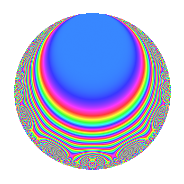# Properties

 Label 402.2.aLevel 402 Weight 2 Character orbit a Rep. character $$\chi_{402}(1,\cdot)$$ Character field $$\Q$$ Dimension 11 Newform subspaces 7 Sturm bound 136 Trace bound 5

# Related objects

## Defining parameters

 Level: $$N$$ = $$402 = 2 \cdot 3 \cdot 67$$ Weight: $$k$$ = $$2$$ Character orbit: $$[\chi]$$ = 402.a (trivial) Character field: $$\Q$$ Newform subspaces: $$7$$ Sturm bound: $$136$$ Trace bound: $$5$$ Distinguishing $$T_p$$: $$5$$, $$7$$

## Dimensions

The following table gives the dimensions of various subspaces of $$M_{2}(\Gamma_0(402))$$.

Total New Old
Modular forms 72 11 61
Cusp forms 65 11 54
Eisenstein series 7 0 7

The following table gives the dimensions of the cuspidal new subspaces with specified eigenvalues for the Atkin-Lehner operators and the Fricke involution.

$$2$$$$3$$$$67$$FrickeDim.
$$+$$$$+$$$$+$$$$+$$$$1$$
$$+$$$$+$$$$-$$$$-$$$$2$$
$$+$$$$-$$$$+$$$$-$$$$1$$
$$+$$$$-$$$$-$$$$+$$$$1$$
$$-$$$$+$$$$+$$$$-$$$$3$$
$$-$$$$-$$$$-$$$$-$$$$3$$
Plus space$$+$$$$2$$
Minus space$$-$$$$9$$

## Trace form

 $$11q + q^{2} - q^{3} + 11q^{4} + 6q^{5} + q^{6} + 4q^{7} + q^{8} + 11q^{9} + O(q^{10})$$ $$11q + q^{2} - q^{3} + 11q^{4} + 6q^{5} + q^{6} + 4q^{7} + q^{8} + 11q^{9} + 6q^{10} + 4q^{11} - q^{12} - 10q^{13} - 2q^{15} + 11q^{16} - 2q^{17} + q^{18} - 4q^{19} + 6q^{20} - 4q^{21} + 4q^{22} + 12q^{23} + q^{24} + 25q^{25} + 14q^{26} - q^{27} + 4q^{28} + 2q^{29} + 2q^{30} - 12q^{31} + q^{32} + 4q^{33} + 2q^{34} - 8q^{35} + 11q^{36} - 2q^{37} - 4q^{38} - 14q^{39} + 6q^{40} - 6q^{41} + 4q^{42} - 4q^{43} + 4q^{44} + 6q^{45} - 20q^{47} - q^{48} - 9q^{49} - q^{50} - 10q^{51} - 10q^{52} + 22q^{53} + q^{54} - 24q^{55} - 12q^{57} + 10q^{58} - 24q^{59} - 2q^{60} - 26q^{61} - 24q^{62} + 4q^{63} + 11q^{64} - 12q^{65} - 12q^{66} + q^{67} - 2q^{68} - 16q^{69} - 32q^{70} - 28q^{71} + q^{72} + 34q^{73} + 14q^{74} - 15q^{75} - 4q^{76} - 16q^{77} - 14q^{78} - 20q^{79} + 6q^{80} + 11q^{81} - 6q^{82} + 24q^{83} - 4q^{84} - 12q^{85} - 12q^{86} + 6q^{87} + 4q^{88} - 18q^{89} + 6q^{90} - 24q^{91} + 12q^{92} - 4q^{93} - 32q^{94} - 56q^{95} + q^{96} - 10q^{97} - 7q^{98} + 4q^{99} + O(q^{100})$$

## Decomposition of $$S_{2}^{\mathrm{new}}(\Gamma_0(402))$$ into newform subspaces

Label Dim. $$A$$ Field CM Traces A-L signs $q$-expansion
$$a_2$$ $$a_3$$ $$a_5$$ $$a_7$$ 2 3 67
402.2.a.a $$1$$ $$3.210$$ $$\Q$$ None $$-1$$ $$-1$$ $$1$$ $$-3$$ $$+$$ $$+$$ $$+$$ $$q-q^{2}-q^{3}+q^{4}+q^{5}+q^{6}-3q^{7}+\cdots$$
402.2.a.b $$1$$ $$3.210$$ $$\Q$$ None $$-1$$ $$1$$ $$-3$$ $$-1$$ $$+$$ $$-$$ $$-$$ $$q-q^{2}+q^{3}+q^{4}-3q^{5}-q^{6}-q^{7}+\cdots$$
402.2.a.c $$1$$ $$3.210$$ $$\Q$$ None $$-1$$ $$1$$ $$2$$ $$0$$ $$+$$ $$-$$ $$+$$ $$q-q^{2}+q^{3}+q^{4}+2q^{5}-q^{6}-q^{8}+\cdots$$
402.2.a.d $$1$$ $$3.210$$ $$\Q$$ None $$1$$ $$-1$$ $$2$$ $$2$$ $$-$$ $$+$$ $$+$$ $$q+q^{2}-q^{3}+q^{4}+2q^{5}-q^{6}+2q^{7}+\cdots$$
402.2.a.e $$2$$ $$3.210$$ $$\Q(\sqrt{3})$$ None $$-2$$ $$-2$$ $$0$$ $$6$$ $$+$$ $$+$$ $$-$$ $$q-q^{2}-q^{3}+q^{4}+2\beta q^{5}+q^{6}+(3+\cdots)q^{7}+\cdots$$
402.2.a.f $$2$$ $$3.210$$ $$\Q(\sqrt{41})$$ None $$2$$ $$-2$$ $$1$$ $$-1$$ $$-$$ $$+$$ $$+$$ $$q+q^{2}-q^{3}+q^{4}+\beta q^{5}-q^{6}-\beta q^{7}+\cdots$$
402.2.a.g $$3$$ $$3.210$$ 3.3.316.1 None $$3$$ $$3$$ $$3$$ $$1$$ $$-$$ $$-$$ $$-$$ $$q+q^{2}+q^{3}+q^{4}+(1-\beta _{2})q^{5}+q^{6}+\cdots$$

## Decomposition of $$S_{2}^{\mathrm{old}}(\Gamma_0(402))$$ into lower level spaces

$$S_{2}^{\mathrm{old}}(\Gamma_0(402)) \cong$$ $$S_{2}^{\mathrm{new}}(\Gamma_0(67))$$$$^{\oplus 4}$$$$\oplus$$$$S_{2}^{\mathrm{new}}(\Gamma_0(134))$$$$^{\oplus 2}$$$$\oplus$$$$S_{2}^{\mathrm{new}}(\Gamma_0(201))$$$$^{\oplus 2}$$